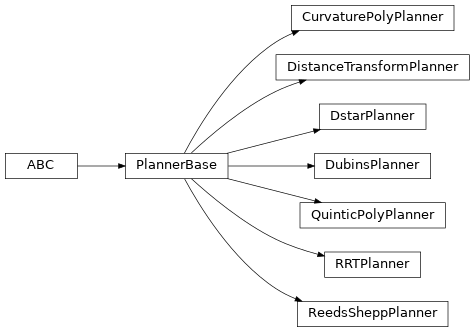# Path planning

A set of path planners for robots operating in planar environments with configuration $$\vec{q} \in \mathbb{R}^2$$ or $$\vec{q} \in \mathbb{R}^2 \times S^1 \sim \SE{2}$$. All inherit from PlannerBase.

Some planners are based on code from the PathPlanning category of PythonRobotics by Atsushi Sakai.Planner

Plans in

Discrete/Continuous

Obstacle avoidance

Bug2

$$\mathbb{R}^2$$

discrete

yes

DistanceTransformPlanner

$$\mathbb{R}^2$$

discrete

yes

DstarPlanner

$$\mathbb{R}^2$$

discrete

yes

PRMPlanner

$$\mathbb{R}^2$$

continuous

yes

LatticePlanner

$$\SE{2}$$

discrete

yes

DubinsPlanner

$$\SE{2}$$

continuous

no

ReedsSheppPlanner

$$\SE{2}$$

continuous

no

CurvaturePolyPlanner

$$\SE{2}$$

continuous

no

QuinticPolyPlanner

$$\SE{2}$$

continuous

no

RRTPlanner

$$\SE{2}$$

continuous

yes